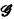Home

# On the undecidability of finite planar cubic graphs1

## Extract

In the March, 1971 issue of this Journal  a paper of ours was published purporting to prove the hereditary undecidability of the first-order theory of finite planar graphs. The proof presented there contains an error which is unfortunately “unfixable” by the methods of that paper. The theorem however is true and we demonstrate here a generalization to finite cubic (exactly three edges at each vertex) planar graphs. The method involves coding the halting problem for a Turing machine into the theory of these graphs by considering special printouts of computations. Let us first consider a discussion of the aforementioned mistake and see what can be learned from it.

By a graphwe will mean a nonempty set V of points together with a set I of unordered pairs of points of V. Each point i = {u, v) ∈ I is an edge ofA graph is called finite if ∣V∣ is finite. A graph is said to be planar iff it can be embedded in the plane (i.e., drawn in the plane so that no two edges intersect).

In the earlier paper the method of proof was a semantic embedding of certain binary relations into finite planar graphs. The essential idea was, for a given relation, to let the vertices of the graph interpret the field of the relation and the edges represent the related pairs. This method works for arbitrary graphs, but in the planar case has two main difficulties.

## Footnotes

Hide All
1

The authors would like to thank Professor Michael Morley for his many helpful conversations which led to the preparation of this paper.

Hide All

# On the undecidability of finite planar cubic graphs1

## Metrics

### Full text viewsFull text views reflects the number of PDF downloads, PDFs sent to Google Drive, Dropbox and Kindle and HTML full text views.

Total number of HTML views: 0
Total number of PDF views: 0 *Loading metrics...

### Abstract viewsAbstract views reflect the number of visits to the article landing page.

Total abstract views: 0 *Loading metrics...

* Views captured on Cambridge Core between <date>. This data will be updated every 24 hours.

Usage data cannot currently be displayed